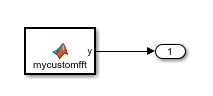## Synchronize Multithreaded FFTW Planning in Code Generated from a MATLAB Function Block

This example shows how to generate code that synchronizes multithreaded access to the FFTW planning process for FFTW library calls in code generated from a MATLAB Function block.

The code generator produces FFTW library calls when all of these conditions are true:

If you integrate the code that contains the FFTW calls with external code that runs on multiple threads, then you must prevent concurrent access to the FFTW planning process. In your FFT library callback class, implement the `lock` and `unlock` methods. You must also provide C code that manages a lock or mutex. Many libraries, such as OpenMP, pthreads, and the C++ standard library (C++ 11 and later), provide locks. This example shows how to implement the `lock` and `unlock` methods and provide supporting C code. To manage a lock, this example uses the OpenMP library.

### Prerequisites

Before you start, for the basic workflow for generating FFTW library calls for fast Fourier transforms in a MATLAB Function block, see Speed Up Fast Fourier Transforms in Code Generated from a MATLAB Function Block.

You must have:

• Access to an installed FFTW library.

• A compiler that supports the OpenMP library. To use a different library, such as pthreads, modify the supporting C code accordingly.

### Create a Model with a MATLAB Function Block That Calls an FFT Function

1. Create a Simulink® model and add a MATLAB Function block to it.

2. Add this code to the MATLAB Function block.

```function y = mycustomfft() t = 0:1/50:10-1/50; x = sin(2*pi*15*t) + sin(2*pi*20*t); y = fft(x); for k = 1:100 y = y + ifft(x+k); end ```

3. Add an `outport` block and connect it to the MATLAB Function block.### Write Supporting C Code

Write C functions that initialize, set, and unset a lock. This example uses the OpenMP library to manage the lock. For a different library, modify the code accordingly.

• Create a file `mylock.c` that contains this C code:

```#include "mylock.h" #include "omp.h" static omp_nest_lock_t lockVar; void mylock_initialize(void) { omp_init_nest_lock(&lockVar); } void mylock(void) { omp_set_nest_lock(&lockVar); } void myunlock(void) { omp_unset_nest_lock(&lockVar); } ```

• Create a header file `mylock.h` that contains:

```#ifndef MYLOCK_H #define MYLOCK_H void mylock_initialize(void); void mylock(void); void myunlock(void); #endif```

### Create an FFT Library Callback Class

Write an FFT callback class `myfftcb` that:

• Specifies the FFTW library.

• Implements `lock` and `unlock` methods that call the supporting C code to control access to the FFTW planning.

Use this class as a template. Replace `fftwLocation` with the location of your FFTW library installation.

```classdef myfftcb < coder.fftw.StandaloneFFTW3Interface methods (Static) function th = getNumThreads coder.inline('always'); th = int32(coder.const(1)); end function lock() coder.cinclude('mylock.h', 'InAllSourceFiles', true); coder.inline('always'); coder.ceval('mylock'); end function unlock() coder.cinclude('mylock.h', 'InAllSourceFiles', true); coder.inline('always'); coder.ceval('myunlock'); end function updateBuildInfo(buildInfo, ctx) fftwLocation = '\usr\lib\fftw'; includePath = fullfile(fftwLocation, 'include'); buildInfo.addIncludePaths(includePath); libPath = fullfile(fftwLocation, 'lib'); %Double libName1 = 'libfftw3-3'; [~, libExt] = ctx.getStdLibInfo(); libName1 = [libName1 libExt]; addLinkObjects(buildInfo, libName1, libPath, 1000, true, true); %Single libName2 = 'libfftw3f-3'; [~, libExt] = ctx.getStdLibInfo(); libName2 = [libName2 libExt]; addLinkObjects(buildInfo, libName2, libPath, 1000, true, true); end end end ```

### Configure Code Generation Parameters and Build the Model

1. Configure code generation to use the FFTW callback class and the C code called by the `lock` and `unlock` methods. Configure code generation to generate a call to `mylock_initialize` in the initialization code.

In the Configuration Parameters dialog box:

• Set Custom FFT library callback to `myfftcb`.

• In Code Generation > Custom Code, under Additional build information, set Source files to `mylock.c`.

• In Code Generation > Custom Code, under Insert custom C code in generated, set Initialize function to `mylock_initialize();`.

2. Build the model.